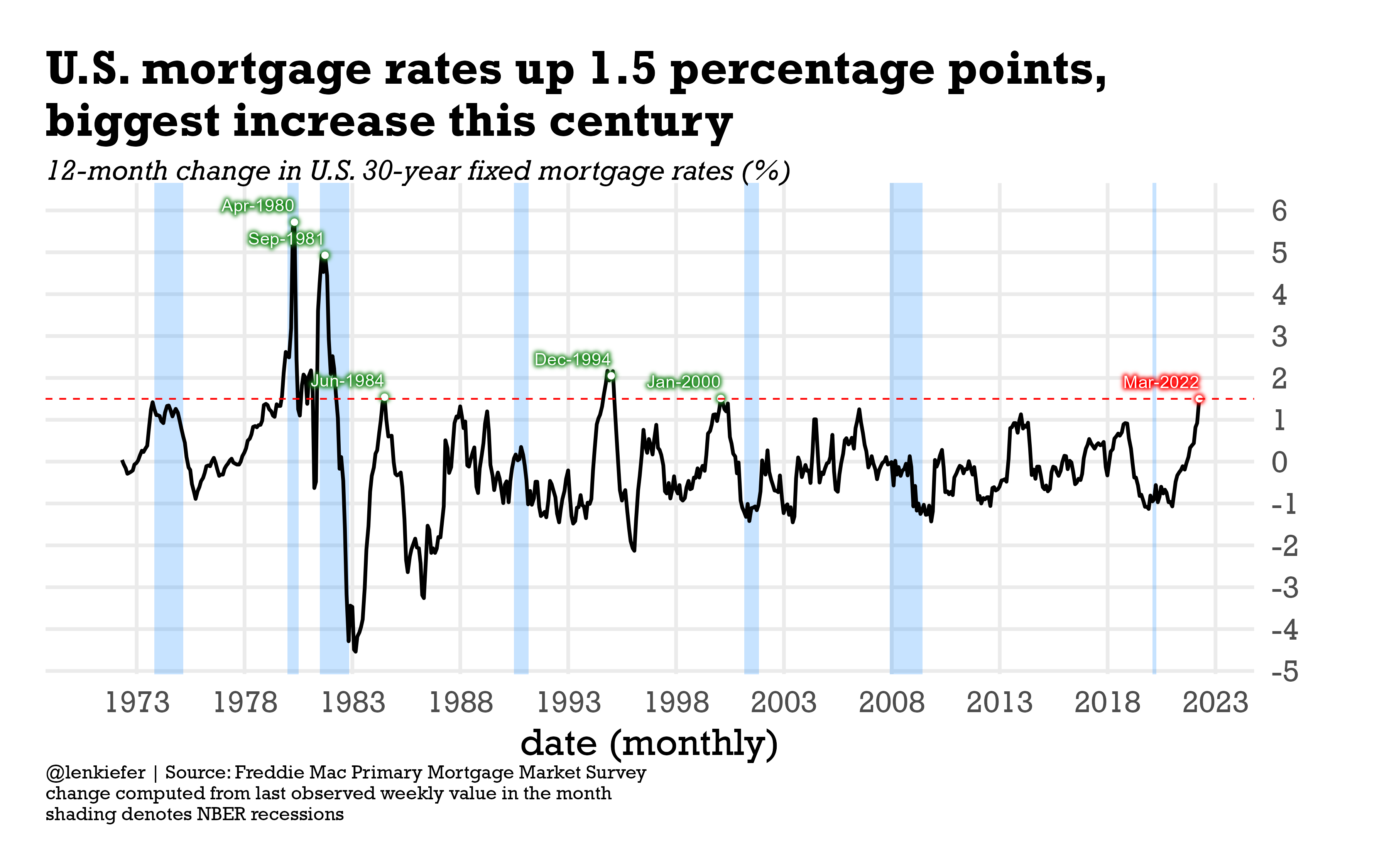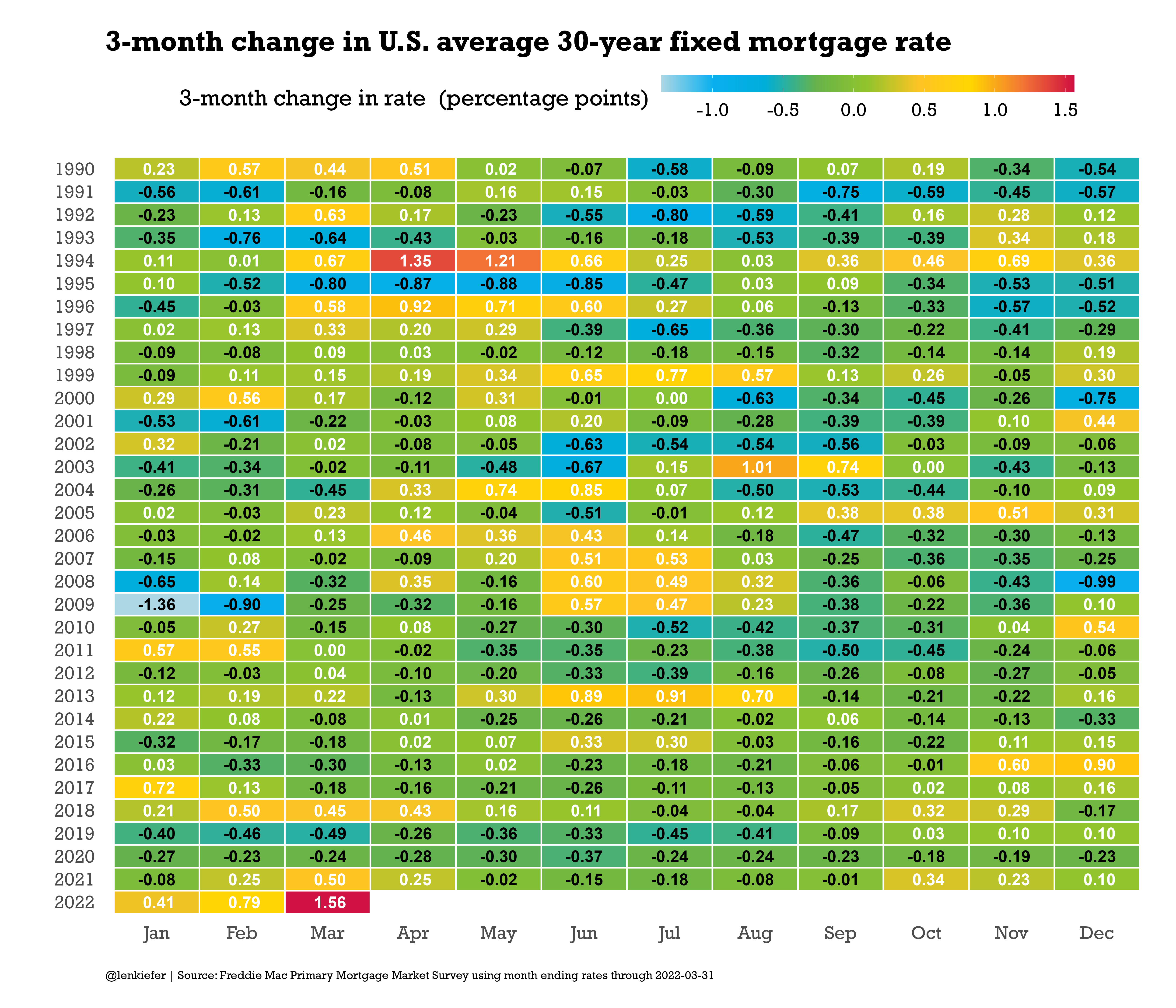# Mortgage rate increase

## Charting the largest increase in mortgage rates this century

Mortgage rates have been increasing. If you measure rates with the last observed value in the month, then March 2022 had the largest 12-month increase of the U.S. average 30-year fixed mortgage rate in the 21st century of 1.5 percentage points. If you go back to the prior century there were five periods where rates increased by at least 1.5 percentage points on a 12-month basis.

Of those periods, two were during a recession, while for the other three, the economy avoided a recession for at least the next 12 months. For a related chart, see Calculated Risk, which charts the percent change in mortgage rates. Coming off a very low base, the recent increases are the near largest recorded.

Let’s make a couple charts with R

First we’ll make this chart, same as the tweet.Then we’ll make another one focusing on 3-month changes.# Code for line chart

The first chart uses just a touch of ggfx to highlight some of the points.

################################
################################

library(ggfx)
library(tidyverse)
library(data.table)
library(extrafont)

################################
# create chart theme----
################################

theme_len <- function(font="Arial", bs=24,...){
theme_minimal(base_size=bs,base_family=font,...) %+replace%
theme(plot.caption=element_text(hjust=0,size=rel(0.5)),
plot.title=element_text(size=rel(1.25),face="bold",hjust=0,
margin=margin(0,0,10,0)),
plot.subtitle=element_text(face="italic",hjust=0,margin=margin(0,0,10,0)
,size=rel(0.75)
),
plot.background=element_rect(fill="white",color=NA),
panel.background=element_rect(fill="white",color=NA),
plot.margin=margin(1,1,1,1,"cm")
)

}

################################
################################

# nber recessions data frame just since 1973

"Peak, Trough
1973-11-01, 1975-03-01
1980-01-01, 1980-07-01
1981-07-01, 1982-11-01
1990-07-01, 1991-03-01
2001-03-01, 2001-11-01
2007-12-01, 2009-06-01
2020-02-02, 2020-04-01"), sep=',',

# mortgage data
df$date <- as.Date(df$date,format="%m/%d/%Y")
dd <- last(df\$date)

dfm3 <-
df %>% group_by(year=year(date),
month=month(date),
monthf=fct_reorder(format(date,"%b"),month(date)),
month=as.factor(month(date))) %>%
summarize(rate=last(pmms30,na.rm=TRUE),
date= last(date,na.rm=TRUE),
rate15=mean(pmms15,na.rm=TRUE)) %>%
ungroup()%>%
mutate(dr=rate-lag(rate,3),
dr12=rate-lag(rate,12))

# create indicators for peaks

dfm3d <- filter(dfm3,date %in% as.Date(c("2022-03-31",
"2000-01-28",
"1994-12-30",
"1984-06-29",
"1981-09-25",
"1980-04-25")))

# create plot

ggplot(data=dfm3, aes(x=date,y=dr12, label=format(date,"%b-%Y")))+
geom_rect(data=filter(recessions.df,Peak>="1970-01-01"),
inherit.aes=FALSE,aes(xmin=Peak,xmax=Trough,ymin=-Inf,ymax=Inf),
fill="dodgerblue",alpha=0.25)+
geom_line(size=1.1)+
theme_len(font="Rockwell")+
scale_x_date(date_breaks="5 years",date_labels="%Y")+
with_outer_glow(geom_point(data=.%>% filter(date==max(date)), color="white"),colour="red",sigma=5,expand=5)+
with_outer_glow(geom_text(data= tail(dfm3d,1), color="white",nudge_y=0.4,hjust=1),colour="red",sigma=5,expand=5)+
geom_hline(data=.%>% filter(date==max(date)), aes(yintercept=dr12),linetype=2,color="red")+
theme(panel.grid.minor=element_blank())+
scale_y_continuous(position="right",breaks=seq(-5,6,1))+
labs(x="date (monthly)",
y="",
title="U.S. mortgage rates up 1.5 percentage points,\nbiggest increase this century",
subtitle="12-month change in U.S. 30-year fixed mortgage rates (%)",
caption="@lenkiefer | Source: Freddie Mac Primary Mortgage Market Survey\nchange computed from last observed weekly value in the month\nshading denotes NBER recessions")

## Code for tile plot

And here’s the code for the tile plot. The code to construct the color scheme is described in this post Housing usually heats up in summer.

# Function for colors ----
# adapted from https://drsimonj.svbtle.com/creating-corporate-colour-palettes-for-ggplot2
#####################################################################################
## Make Color Scale ----  ##
#####################################################################################
my_colors <- c(
"green"      = rgb(103,180,75, maxColorValue = 256),
"green2"      = rgb(147,198,44, maxColorValue = 256),
"lightblue"  =  rgb(9, 177,240, maxColorValue = 256),
"lightblue2" = rgb(173,216,230, maxColorValue = 256),
'blue'       = "#00aedb",
'red'        = "#d11141",
'orange'     = "#f37735",
'yellow'     = "#ffc425",
'gold'       = "#FFD700",
'light grey' = "#cccccc",
'purple'     = "#551A8B",
'dark grey'  = "#8c8c8c")

my_cols <- function(...) {
cols <- c(...)
if (is.null(cols))
return (my_colors)
my_colors[cols]
}

my_palettes <- list(
main  = my_cols("blue", "green", "yellow"),
cool  = my_cols("blue", "green"),
cool2hot = my_cols("lightblue2","lightblue", "blue","green", "green2","yellow","gold", "orange", "red"),
hot   = my_cols("yellow", "orange", "red"),
mixed = my_cols("lightblue", "green", "yellow", "orange", "red"),
mixed2 = my_cols("lightblue2","lightblue", "green", "green2","yellow","gold", "orange", "red"),
mixed3 = my_cols("lightblue2","lightblue", "green", "yellow","gold", "orange", "red"),
mixed4 = my_cols("lightblue2","lightblue", "green", "green2","yellow","gold", "orange", "red","purple"),
mixed5 = my_cols("lightblue","green", "green2","yellow","gold", "orange", "red","purple","blue"),
mixed6 = my_cols("green", "gold", "orange", "red","purple","blue"),
grey  = my_cols("light grey", "dark grey")
)

my_pal <- function(palette = "main", reverse = FALSE, ...) {
pal <- my_palettes[[palette]]

if (reverse) pal <- rev(pal)

colorRampPalette(pal, ...)
}

scale_color_mycol <- function(palette = "main", discrete = TRUE, reverse = FALSE, ...) {
pal <- my_pal(palette = palette, reverse = reverse)

if (discrete) {
discrete_scale("colour", paste0("my_", palette), palette = pal, ...)
} else {
scale_color_gradientn(colours = pal(256), ...)
}
}

scale_fill_mycol <- function(palette = "main", discrete = TRUE, reverse = FALSE, ...) {
pal <- my_pal(palette = palette, reverse = reverse)

if (discrete) {
discrete_scale("fill", paste0("my_", palette), palette = pal, ...)
} else {
scale_fill_gradientn(colours = pal(256), ...)
}
}

# Make chart ----
df %>% group_by(year=year(date),
month=month(date),
monthf=fct_reorder(format(date,"%b"),month(date)),
month=as.factor(month(date))) %>%
summarize(rate=last(pmms30,na.rm=TRUE),
rate15=mean(pmms15,na.rm=TRUE)) %>%
ungroup()%>%
mutate(dr=rate-lag(rate,3)) %>%
filter(year>1989) %>%
ggplot(aes(x=monthf,y=fct_reorder(factor(year),-year),
fill=dr,
label=format(round(dr,2)),small=2))+

geom_tile()+
geom_tile(fill=NA,color="white",size=0.75)+
geom_text(aes(color=ifelse(dr<0,"black","white")),
size=6,fontface="bold",font="Rockwell",show.legend="false")+
scale_color_manual(values=c("black","white"))+
theme_len(font="Rockwell")+
theme(plot.caption=element_text(hjust=0),
panel.grid=element_blank(),
legend.key.width=unit(3,"cm"),
legend.position="top")+
scale_fill_mycol(palette="cool2hot",name="3-month change in rate  (percentage points)",discrete=FALSE)+
labs(x="",y="",
title="3-month change in U.S. average 30-year fixed mortgage rate",
caption=paste0("@lenkiefer | Source: Freddie Mac Primary Mortgage Market Survey using month ending rates through ",
dd))